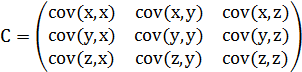• ## 协方差的Matlab实现

万次阅读 2018-06-07 16:54:29
Matlab协方差实战 上面涉及的内容都比较容易，协方差矩阵似乎也很简单，但实战起来就很容易让人迷茫了。必须要明确一点， 协方差矩阵计算的是不同维度之间的协方差，而不是不同样本之间的。 这个我将结合下面的例子...
学过概率统计的孩子都知道，统计里最基本的概念就是样本的均值，方差，或者再加个标准差。首先我们给你一个含有n个样本的集合，依次给出这些概念的公式描述，这些高中学过数学的孩子都应该知道吧，一带而过。很显然，均值描述的是样本集合的中间点，它告诉我们的信息是很有限的，而标准差给我们描述的则是样本集合的各个样本点到均值的距离之平均。以这两个集合为例，[0，8，12，20]和[8，9，11，12]，两个集合的均值都是10，但显然两个集合差别是很大的，计算两者的标准差，前者是8.3，后者是1.8，显然后者较为集中，故其标准差小一些，标准差描述的就是这种“散布度”。之所以除以n-1而不是除以n，是因为这样能使我们以较小的样本集更好的逼近总体的标准差，即统计上所谓的“无偏估计”。而方差则仅仅是标准差的平方。为什么需要协方差？上面几个统计量看似已经描述的差不多了，但我们应该注意到，标准差和方差一般是用来描述一维数据的，但现实生活我们常常遇到含有多维数据的数据集，最简单的大家上学时免不了要统计多个学科的考试成绩。面对这样的数据集，我们当然可以按照每一维独立的计算其方差，但是通常我们还想了解更多，比如，一个男孩子的猥琐程度跟他受女孩子欢迎程度是否存在一些联系啊，嘿嘿~协方差就是这样一种用来度量两个随机变量关系的统计量，我们可以仿照方差的定义：来度量各个维度偏离其均值的程度，标准差可以这么来定义：协方差的结果有什么意义呢？如果结果为正值，则说明两者是正相关的(从协方差可以引出“相关系数”的定义)，也就是说一个人越猥琐就越受女孩子欢迎，嘿嘿，那必须的~结果为负值就说明负相关的，越猥琐女孩子越讨厌，可能吗？如果为0，也是就是统计上说的“相互独立”。从协方差的定义上我们也可以看出一些显而易见的性质，如：协方差多了就是协方差矩阵上一节提到的猥琐和受欢迎的问题是典型二维问题，而协方差也只能处理二维问题，那维数多了自然就需要计算多个协方差，比如n维的数据集就需要计算 n! / ((n-2)!*2) 个协方差，那自然而然的我们会想到使用矩阵来组织这些数据。给出协方差矩阵的定义：这个定义还是很容易理解的，我们可以举一个简单的三维的例子，假设数据集有三个维度，则协方差矩阵为可见，协方差矩阵是一个对称的矩阵，而且对角线是各个维度上的方差。Matlab协方差实战上面涉及的内容都比较容易，协方差矩阵似乎也很简单，但实战起来就很容易让人迷茫了。必须要明确一点，协方差矩阵计算的是不同维度之间的协方差，而不是不同样本之间的。这个我将结合下面的例子说明，以下的演示将使用Matlab，为了说明计算原理，不直接调用Matlab的cov函数(蓝色部分为Matlab代码)。首先，随机产生一个10*3维的整数矩阵作为样本集，10为样本的个数，3为样本的维数。mysample = fix(rand(10,3)*50)根据公式，计算协方差需要计算均值，那是按行计算均值还是按列呢，我一开始就老是困扰这个问题。前面我们也特别强调了，协方差矩阵是计算不同维度间的协方差，要时刻牢记这一点。样本矩阵的每行是一个样本，每列为一个维度，所以我们要按列计算均值。为了描述方便，我们先将三个维度的数据分别赋值：>> dim1 = mysample(:,1);
>> dim2 = mysample(:,2);
>> dim3 = mysample(:,3);计算dim1与dim2，dim1与dim3，dim2与dim3的协方差：>> sum((dim1 - mean(dim1)) .* (dim2 - mean(dim2))) / (size(mysample, 1) - 1)  %得到 -147.0667
>> sum((dim1 - mean(dim1)) .* (dim3 - mean(dim3))) / (size(mysample, 1) - 1)  %得到  -82.2667
>> sum((dim2 - mean(dim2)) .* (dim3 - mean(dim3))) / (size(mysample, 1) - 1)  %得到   76.5111搞清楚了这个后面就容易多了，协方差矩阵的对角线就是各个维度上的方差，下面我们依次计算：>> var(dim1)  %得到 227.8778
>> var(dim2)  %得到 179.8222
>> var(dim3)  %得到 156.7111 这样，我们就得到了计算协方差矩阵所需要的所有数据，调用Matlab自带的cov函数进行验证：>> cov(mysample)把我们计算的数据对号入座，是不是一摸一样？Update今天突然发现，原来协方差矩阵还可以这样计算，先让样本矩阵中心化，即每一维度减去该维度的均值，使每一维度上的均值为0，然后直接用新的到的样本矩阵乘上它的转置，然后除以(N-1)即可。其实这种方法也是由前面的公式推导而来，只不过理解起来不是很直观，但在抽象的公式推导时还是很常用的！同样给出Matlab代码实现：>> temp = mysample - repmat(mean(mysample), 10, 1);
>> result = temp' * temp ./ (size(mysample, 1) - 1)总结理解协方差矩阵的关键就在于牢记它计算的是不同维度之间的协方差，而不是不同样本之间，拿到一个样本矩阵，我们最先要明确的就是一行是一个样本还是一个维度，心中明确这个整个计算过程就会顺流而下，这么一来就不会迷茫了~ 
展开全文• 本文档中，从实用的角度详细讲解了协方差的概念、用法及代码实现。是我经过查阅资料，实践验证后的详细资料，希望对初接触协方差的同学有帮助。有详细的例子，第一次上次文档，希望大家支持下 O(∩_∩)O~
• 协方差就是这样一种用来度量两个随机变量关系的统计量，我们可以仿照方差的定义：来度量各个维度偏离其均值的程度，标准差可以这么来定义：从协方差的定义上我们也可以看出一些显而易见的性质，如：协方差多了就是...
(参考文章)标准差和方差一般是用来描述一维数据的所谓的维数，拿EEG信号来说，每个通道就是一个维度，而同一个通道的每个一数字是样本。协方差就是这样一种用来度量两个随机变量关系的统计量，我们可以仿照方差的定义：来度量各个维度偏离其均值的程度，标准差可以这么来定义：从协方差的定义上我们也可以看出一些显而易见的性质，如：协方差多了就是协方差矩阵这个定义还是很容易理解的，我们可以举一个简单的三维的例子，假设数据集有三个维度，则协方差矩阵为其中cov(x,y)就是一个数，对应x,y渠道平均值后，求内积再求平均值，就是x,y之间的协方差可见，协方差矩阵是一个对称的矩阵，而且对角线是各个维度上的方差。Matlab协方差实战协方差矩阵计算的是不同维度之间的协方差，而不是不同样本之间的。这个我将结合下面的例子说明，以下的演示将使用Matlab，为了说明计算原理，不直接调用Matlab的cov函数 。首先，随机产生一个10*3维的整数矩阵作为样本集，10为样本的个数，3为样本的维数。1MySample = fix(rand(10,3)*50)根据公式，计算协方差需要计算均值，那是按行计算均值还是按列呢，我一开始就老是困扰这个问题。前面我们也特别强调了，协方差矩阵是计算不同维度间的协方差，要时刻牢记这一点。样本矩阵的每行是一个样本，每列为一个维度，所以我们要按列计算均值。dim1 = MySample(:,1);dim2 = MySample(:,2);dim3 = MySample(:,3);计算dim1与dim2，dim1与dim3，dim2与dim3的协方差：sum( (dim1-mean(dim1)) .* (dim2-mean(dim2)) ) / ( size(MySample,1)-1 ) % 得到  74.5333sum( (dim1-mean(dim1)) .* (dim3-mean(dim3)) ) / ( size(MySample,1)-1 ) % 得到  -10.0889sum( (dim2-mean(dim2)) .* (dim3-mean(dim3)) ) / ( size(MySample,1)-1 ) % 得到  -106.4000搞清楚了这个后面就容易多了，协方差矩阵的对角线就是各个维度上的方差，下面我们依次计算：std(dim1)^2 % 得到   108.3222 std()是求标准差的函数std(dim2)^2 % 得到   260.6222std(dim3)^2 % 得到   94.1778这样，我们就得到了计算协方差矩阵所需要的所有数据，调用Matlab自带的cov函数进行验证：cov(MySample)=简化算法：由于分别为m 与n 个标量元素的列向量随机变量X 与Y，二者对应的期望值分别为μ与ν，这两个变量之间的协方差定义为m×n矩阵也就是说，先让样本矩阵中心化，即每一维度减去该维度的均值，使每一维度上的均值为0，然后直接用新得到的样本矩阵乘上它的转置，然后除以(N-1)即可。即为上式。N 表示的是每一维度的样本数。例子：X’=[1 2 3 ] %均值是2Y’=[4 5 6] %均值是5则会得到 x-2=[-1 0 1]’ ; y-5=[-1 0 1]’进行转置相乘后 求均值则得到 ((x-2)’*(y-5))/2=[1 1;1 1] 同结果是一样的再举个例子：a=fix(rand(10,3)*50)a =37 42 1712 12 4125 40 2934 12 2744 46 4547 17 1427 9 376 12 377 30 1912 23 28x=a-repmat(mean(a),10,1)%这里mean(a)得到的是列平均值，repmat(mean(a),10,1)是产生一个10行一列的矩阵。在这里的一列是指mean(a)整体x =11.9000 17.7000 -12.4000-13.1000 -12.3000 11.6000-0.1000 15.7000 -0.40008.9000 -12.3000 -2.400018.9000 21.7000 15.600021.9000 -7.3000 -15.40001.9000 -15.3000 7.6000-19.1000 -12.3000 7.6000-18.1000 5.7000 -10.4000-13.1000 -1.3000 -1.4000(x’*x)./ (size(x,1)-1) =232.9889 70.0778 -31.933370.0778 200.6778 -17.2444-31.9333 -17.2444 111.1556
展开全文• z=[1,5;3,4;4,6;5,3]; ...%matlab函数结果 % b=cov(z) %  % 2.9167 -0.8333 % -0.8333 1.6667 %% z_mean=mean(z); result = zeros(2,2); for i = 1:2  for j=1:2  result(i,j)=(z(:,i
z=[1,5;3,4;4,6;5,3];
%%
%matlab函数结果
% b=cov(z)
%
%     2.9167   -0.8333
%    -0.8333    1.6667
%%
z_mean=mean(z);
result = zeros(2,2);
for i = 1:2
for j=1:2
result(i,j)=(z(:,i)-z_mean(i))'*(z(:,j)-z_mean(j));
%result(i,j)=sum((z(:,i)-z_mean(i))'*(z(:,j)-z_mean(j)))/(size(z,1)-1);%用这个公式得到与matlab相同的结果
end
end
%自编函数结果
%     8.7500   -2.5000
%    -2.5000    5.0000

展开全文• 协方差矩阵 协方差也只能处理二维问题，那维数多了自然就需要计算多个协方差，比如n维的数据集就需要计算n!(n−2)!∗2\frac{n!}{(n-2)!*2}个协方差，那自然而然我们会想到使用矩阵来组织这些数据。给出协方差矩阵的...
协方差矩阵

协方差也只能处理二维问题，那维数多了自然就需要计算多个协方差，比如n维的数据集就需要计算n!(n−2)!∗2<!--//--><![CDATA[//><!--
\frac{n!}{(n-2)!*2}
//--><!]]>个协方差，那自然而然我们会想到使用矩阵来组织这些数据。给出协方差矩阵的定义：

Cn∗n=(ci,j,ci,j=cov(Dimi,Dimj))<!--//--><![CDATA[//><!--
C_{n*n}=(c_{i,j}, c_{i,j}=cov(Dim_i, Dim_j))
//--><!]]>这个定义还是很容易理解的，我们可以举一个三维的例子，假设数据集有三个维度，则协方差矩阵为：必须要明确一点，协方差矩阵计算的是不同维度之间的协方差，而不是不同样本之间的。以下的演示将使用Matlab，为了说明计算原理，不直接调用Matlab的cov函数：

首先，随机生成一个10*3维的整数矩阵作为样本集，10为样本的个数，3为样本的维数。

>> mySample=fix(rand(10,3)*50)

mySample =

40     7    32
45    48     1
6    47    42
45    24    46
31    40    33
4     7    37
13    21    37
27    45    19
47    39    32
48    47     8

根据公式，计算协方差需要计算均值，前面特别强调了，协方差矩阵是计算不同维度之间的协方差，要时刻牢记这一点。样本矩阵的每行是一个样本，每列是一个维度，因此我们要按列计算均值。为了描述方便，我们先将三个维度的数据分别赋值：


>> dim1=mySample(:,1)

dim1 =

40
45
6
45
31
4
13
27
47
48

>> dim2=mySample(:,2)

dim2 =

7
48
47
24
40
7
21
45
39
47

>> dim3=mySample(:,3)

dim3 =

32
1
42
46
33
37
37
19
32
8

计算dim1与dim2，dim1与dim3，dim2与dim3的协方差：

>> cov12=sum((dim1-mean(dim1)).*(dim2-mean(dim2)))/(size(mySample,1)-1)

cov12 =

78

>> cov13=sum((dim1-mean(dim1)).*(dim3-mean(dim3)))/(size(mySample,1)-1)

cov13 =

-120.2444

>> cov13=sum((dim1-mean(dim1)).*(dim3-mean(dim3)))/(size(mySample,1)-1)

cov13 =

-120.2444

>> cov13=sum((dim1-mean(dim1)).*(dim3-mean(dim3)))/(size(mySample,1)-1)

cov13 =

-120.2444

>> cov23=sum((dim2-mean(dim2)).*(dim3-mean(dim3)))/(size(mySample,1)-1)

cov23 =

-126.9444


协方差矩阵的对角线上的元素就是各个维度的方差，下面我们依次计算这些方差：

>> var1=std(dim1)^2

var1 =

301.1556

>> var2=std(dim2)^2

var2 =

268.9444

>> var3=std(dim3)^2

var3 =

216.0111

这样，我们就得到了计算协方差矩阵所需要的所有数据，可以调用Matlab的cov函数直接得到协方差矩阵：

>> cov(mySample)

ans =

301.1556   78.0000 -120.2444
78.0000  268.9444 -126.9444
-120.2444 -126.9444  216.0111

计算的结果，和之前的数据填入矩阵后的结果完全相同。
展开全文数据
• ## matlab协方差矩阵

千次阅读 2018-05-29 15:56:28
参考自文章：http://blog.163.com/yuyang_tech/blog/static/216050083201382610424490/cov(X,Y) 求矩阵X与Y的协方差矩阵。...此时，cov(X,Y) 等于cov([X(:) Y(:)])和cov(X(:),Y(:))，即计算两个向量的协方差...
• 最近在论文里看到很多协方差矩阵的表示方式为 Rx=E{XXT} ，但是我们经常用的都时 cov(X)来计算协方差。 其中：X为一维随机序列或者信号的采样， XT 代表 X 的转置序列 设：x=[10 20 32 44 5 7 2 56 7 2]'; 注意有...
• 今天回顾了之前看到的协方差协方差矩阵，发现真的缺漏了很多，所以做如下记录。协方差学过概率统计的应该都知道协方差的定义，接下来就简单说明一下。协方差表示的是两个变量的总体的误差，这与只表示一个变量误差...
• Covariance 翻译为协方差，因此，MATLAB里面的函数cov也就是求协方差了。至于MATLAB语言里面的协方差函数cov的语法是什么样的以及怎么用的，我们稍后再说，这里首先介绍下协方差相关的基础知识点。 本文内容参考自...
• end end c = 10.3333 -4.1667 3.0000 -4.1667 2.3333 -1.5000 3.0000 -1.5000 1.0000 c为求得的协方差矩阵，在matlab以矩阵a的每一列为变量，对应的每一行为样本。这样在矩阵a中就有3个列变量分别为a(:,1), a(:,2),...
• Matlab中cov函数详细解读 １、向量的方差与协方差矩阵 cov(x) 求向量x的方差。 cov(x)为一个数值，数值大小计算公式为S(x)。 cov(x,y) 求向量x与y的协方差矩阵。 cov(x,y)为2*2矩阵， [S(x) C(x,y); ...
• end end c = 10.3333 -4.1667 3.0000 -4.1667 2.3333 -1.5000 3.0000 -1.5000 1.0000 c为求得的协方差矩阵，在matlab以矩阵a的每一列为变量，对应的每一行为样本。这样在矩阵a中就有3个列变量分别为a(:,1), a(:,2),...
• 介绍了Matlab 中如何使用 mean 、var 、std、cov 函数等
• ## 协方差矩阵及matlab实现

万次阅读 多人点赞 2017-09-26 10:26:02
以下的演示将使用Matlab，为了说明计算原理，不直接调用Matlab的cov函数（求协方差矩阵的函数），而是通过列举两个例子来实现： 例1： 首先取 作为计算的例子，接下来给出详细的计算...
• 1. 协方差在统计学的基本概念   统计学里最基本的概念就是样本的均值、方差、标准差。首先，我们给定一个含有n个样本的集合，下面给出这些概念的公式描述：   均值描述的是样本集合的中间点，它告诉我们的...
• 但是在有些用到协方差的算法中， 分母使用了N，而不是N-1。但是由于样本很多，差别不大。 >> v=magic(3) v = 8 1 6 3 5 7 4 9 2 >> cov(v) ans = 7 -8 1 -8 16 -8 ...
• 如果X为矩阵，把矩阵X的行作为观察值，把列作为变量，返回X的协方差矩阵； diag(cov(X))是每列的方差组成的向量； sqrt(diag(cov(X)))是每列的标准差组成的向量； 具体计算如下: S为方差，C为协方差 3.cov(X,...
• 1.matlab的总结 在matlab中调用.m脚本时，只需要像c函数调一样使用.m的文件名。 而在.m文件中作为函数时，加";"和不加";...2.协方差 数学中的方差主要表示两个参数之间的关系，cov越大，表示关系越密
• 说明：这里有n个样本，计算时以n-1代替n是以较小的样本集逼近总体的标准差，即统计上的“无偏估计”，matlab中方差、标准差、协方差计算都是默认n-1，后面会有验证。 仿照方差的定义可以给出协方差的公式： 据......

# 协方差matlabmatlab 订阅